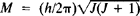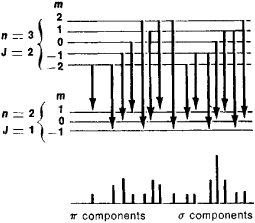# Stark Effect

(redirected from Stark-Lo Surdo effect)
Also found in: Dictionary.

## Stark effect

The effect of an electric field on spectrum lines. The electric field may be externally applied; but in many cases it is an internal field caused by the presence of neighboring ions or atoms in a gas, liquid, or solid. Discovered in 1913 by J. Stark, the effect is most easily studied in the spectra of hydrogen and helium, by observing the light from the cathode dark space of an electric discharge. Because of the large potential drop across this region, the lines are split into several components. For observation perpendicular to the field, the light of these components is linearly polarized.

The linear Stark effect exhibits large, nearly symmetrical patterns. The interpretation of the linear Stark effect was one of the first successes of the quantum theory. According to this theory, the effect of the electric field on the electron orbit is to split each energy level of the principal quantum number n into 2n - 1 equidistant levels, of separation proportional to the field strength. See Atomic structure and spectra

The quadratic Stark effect occurs in lines resulting from the lower energy states of many-electron atoms. The quadratic Stark effect is basic to the explanation of the formation of molecules from atoms, of dielectric constants, and of the broadening of spectral lines.

The intermolecular Stark effect is produced by the action of the electric field from surrounding atoms or ions on the emitting atom. The intermolecular effect causes a shifting and broadening of spectrum lines. The molecules being in motion, these fields are inhomogeneous in space and also in time. Hence the line is not split into resolved components but is merely widened.

The quantum-confined Stark effect is the Stark effect observed in structures in which the hydrogenic system is confined in a layer of thickness much less than its normal diameter. This is not practical with atoms, but the effect is observed with excitons in semiconductor quantum-well heterostructures. It is important that quantum-confined Stark shifts can be much larger than the binding energy of the hydrogenic system. The resulting shifts of the exciton optical absorption lines can be used to make optical beam modulators and self-electrooptic-effect optical switching devices. See Artificially layered structures, Electrooptics, Exciton, Optical modulators, Semiconductor heterostructures

McGraw-Hill Concise Encyclopedia of Physics. © 2002 by The McGraw-Hill Companies, Inc.
The following article is from The Great Soviet Encyclopedia (1979). It might be outdated or ideologically biased.

## Stark Effect

the splitting of spectral lines in electric fields. The effect was discovered in 1913 by J. Stark while studying the spectrum of the hydrogen atom. Observed in the spectra of atoms and of other quantum systems, it results from a shift of a quantum system’s energy levels and the splitting of the energy levels into sublevéis under the influence of an electric field. The splitting and the sublevéis are referred to as Stark splitting and Stark sublevéis. The term “Stark effect” is applied not only to the splitting of spectral lines in electric fields but also to the shift and splitting of energy levels in such fields.Figure 1. Dependence of the magnitude of level splitting A£ on the strength of the electric field E in the linear Stark effect for the splitting into five sub-levels of a hydrogen atom’s energy level specified by a principal quantum number n = 3

The Stark effect has been explained on the basis of quantum mechanics. An atom (or some other quantum system) in a state with a given energy ℰ acquires additional energy Δℰ in an applied electric field E as a result of the polarizability of the atom’s electron shell and the appearance of an induced dipole moment. A nondegenerate energy level, or an energy level to which one possible state of an atom corresponds, will have an energy of ℰ + Δℰ in a field E; that is, the level will shift. The various states of a degenerate energy level may acquire different additional energies Δℰα; here, α = 1, 2, . . . , g, where g is the degree of degeneracy of the level (seeATOM). As a result, a degenerate level is split into Stark sublevéis, the number of which is equal to the number of different values of Δℰα.

Thus, in an electric field, an energy level of an atom with a given angular momentum—where h is Planck’s constant and J = 1, 2, . . . is the quantum number of total angular momentum—is split into sublevéis characterized by different values of the magnetic quantum number mj, which represents the projection of M in the direction of the electric field. The same additional energy Δℰ corresponds to the values –mj and + mj. Therefore, all the Stark sublevéis except the sublevel with m = 0 turn out to have a twofold degeneracy, in contrast to the case of splitting in a magnetic field, where all the sublevéis are not degenerate (seeZEEMAN EFFECT).

A distinction is made between the linear Stark effect, where Δℰ is proportional to E (Figure 1), and the quadratic Stark effect, where Δℰ is proportional to E2 (Figure 2). In the linear effect, the pattern of the energy-level splitting and of the spectral lines produced upon transitions between the levels is symmetric. In the quadratic effect, the pattern is asymmetric.Figure 2. Dependence of the magnitude of level splitting ΔƐ on the strength of the electric field E in the quadratic Stark effect; the sublevéis turn out to be unequally spaced

The linear Stark effect is characteristic of hydrogen in electric fields that are not too strong. For example, in fields of ~104 volts per cm (V/cm), the effect amounts to a few thousandths of an electron volt. An energy level of a hydrogen atom with a given principal quantum number n is split symmetrically into 2n – 1 equidistant sublevéis (Figure 1 corresponds to n = 3, 2n – 1 = 5). The components of a spectral line that is split in a field E are polarized. If the direction of E is perpendicular to an observer, some of the components—called the π components—are polarized parallel to the field, while the remaining components— known as the σ components—are polarized perpendicular to the field. When observed in the direction longitudinal to the field, the π components do not appear and the cr components are replaced by unpolarized components. The intensities of the various components differ. The splitting of the Hα spectral line of hydrogen (the first line of the Balmer series) as a result of the Stark effect in shown in Figure 3.

The linear Stark effect is also observed in hydrogen-like atoms (He +, Li2+, Be3+, . . . ) and for highly excited states of other atoms; in a number of cases, the effect results in the appearance of forbidden lines. The quadratic Stark effect, which exhibits an asymmetric splitting pattern, is typical of many-electron atoms. The magnitude of the quadratic effect is small. For example, in fields of ~105 V/cm, the splitting amounts to a few ten-thousandths of an electron volt. The linear Stark effect is characteristic of molecules that are sufficiently symmetric and have a constant dipole moment. In other cases, the quadratic Stark effect is usually observed.

An important case of the Stark effect is the splitting of the electronic energy levels of an ion in a crystal lattice under the influence of the crystalline field Ecr, which is generated by surrounding ions. Such splitting may be as high as a few hundredths of an electron volt, is taken into account in crystal spectroscopy, and is essential for the operation of quantum mechanical amplifiers.

The Stark effect is also observed in variable electric fields. The change in the position of the Stark sublevéis in a variable field Emay be used to vary the frequency of a quantum transition in quantum-mechanical devices. An example is Stark modulation (seeMICROWAVE SPECTROSCOPY).

In particular, the influence of a rapidly varying electric field on the energy levels of atoms or ions determines the Stark broadeningFigure 3. The splitting of the Hα line of hydrogen in an electric field. Differently polarized line components — the π components and the σ components — appear for specific combinations of sublevéis.

of spectral lines in a plasma. The motion of plasma particles and the associated change in the distances between the particles lead to rapid changes in the electric field around each radiating particle. As a result, the energy levels of atoms or ions shift by differing amounts when they are split, leading to the broadening of spectral lines in the emission spectra of a plasma. Stark broadening makes it possible to estimate the concentration of charged particles in a plasma, for example, in a stellar atmosphere.

### REFERENCES

El’iashevich, M. A. Atomnaia i molekuliarnaia spektroskopiia. Moscow, 1962.
Frish, S. E. Opticheskiespektry atomov. Moscow-Leningrad, 1963.
Townes, C., and A. Shawlow. Radiospektroskopiia. Moscow, 1959. (Translated from English.)

M. A. EL’IASHEVICH